## Monday, May 27, 2019

### MCQ Walkthrough March 2019 9708/32 (India)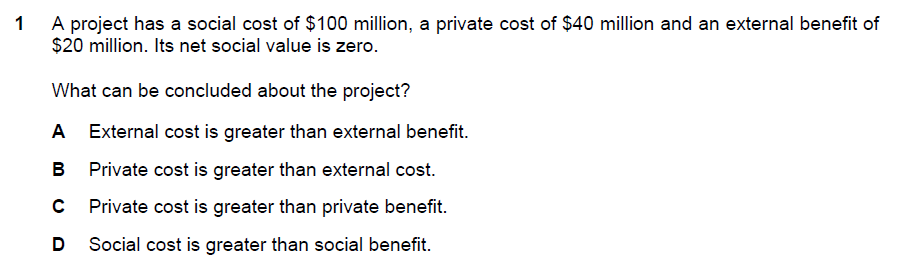SC = \$100 million, PC =  \$40 million and EC = \$ 60 million

SB = \$ 100 million (since net social is 0), EB = \$ 20 million and PB = \$ 80 million. With this information the answer is therefore (A)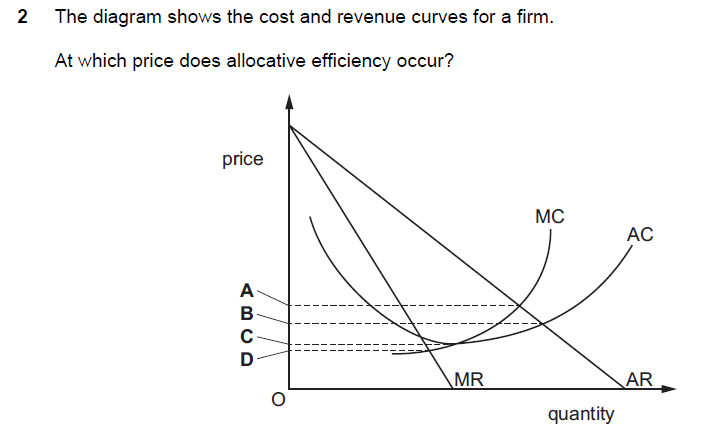Allocative efficiency is when a firm is operating at the level of output where MC = AR. Answer is therefore (A)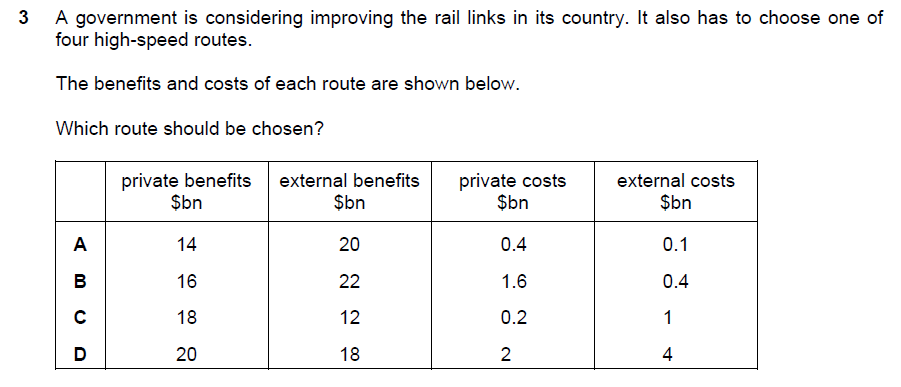The government will always consider a project which delivers the highest net social benefit (SB - SC). The answer is therefore (B) since its NSB = \$36 bn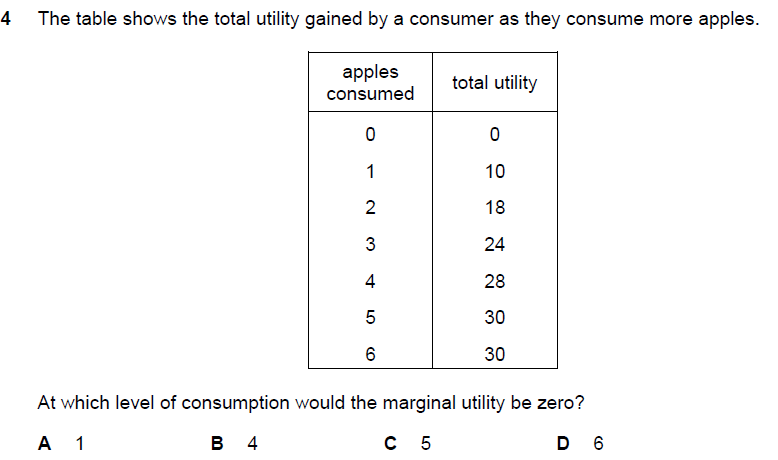Marginal utility = change in TU/ change in Q = (30-30)/ (6-5) = 0. Answer is therefore (D)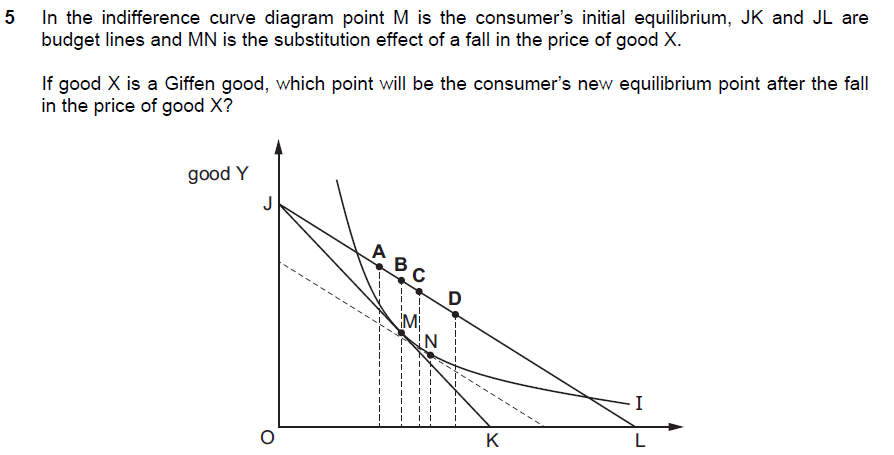For a Giffen good (extreme inferior), its income effect outweighs its substitution effect. In this case, substitution effect would be positive as indicated by a movement from M to N. However, its income effect is so strong that it would eventually offset the initial increase in quantity. Therefore, the answer must be (A) which shows an overall decline in output X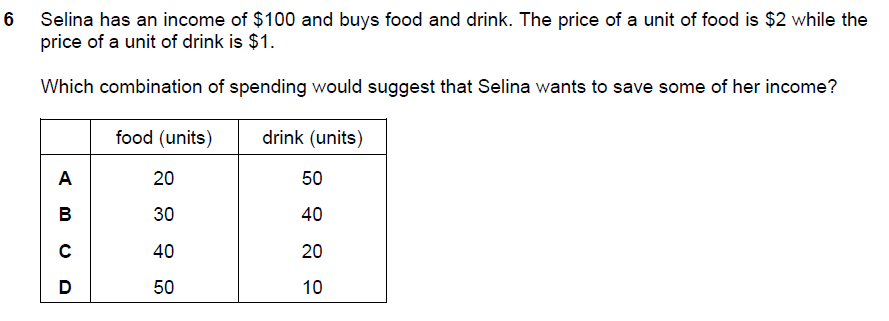20(\$2) + 50(\$1) = \$90. Selina would still have \$10 left for her to save. The answer is A. (B) and (C) indicate that she would spend all her available income. Answer (D) implies that she would overspend her budget by an extra \$10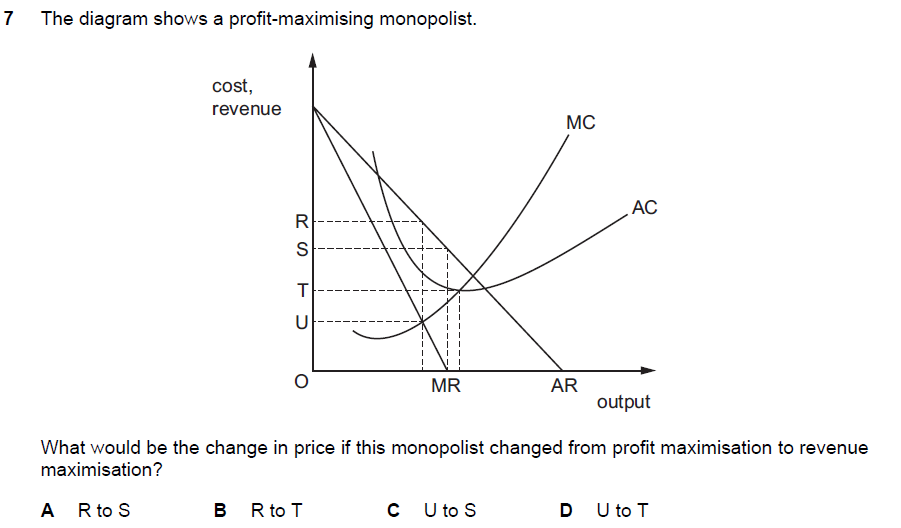The condition for profit maximisation is MC = MR while the condition for revenue maximisation is MR = 0. Therefore it would involve a movement from R to S. Answer is (A)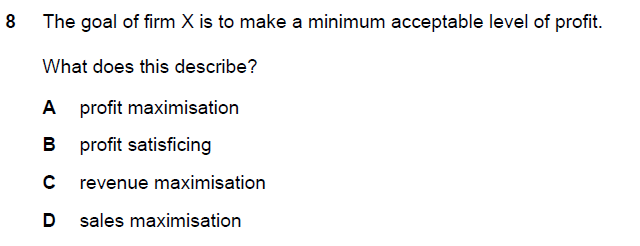This is related to satisficing where business managers attempt to deliver a minimum level of acceptable profit so as to please the shareholders. Meanwhile, it attempts to go for other goals. Answer is (B)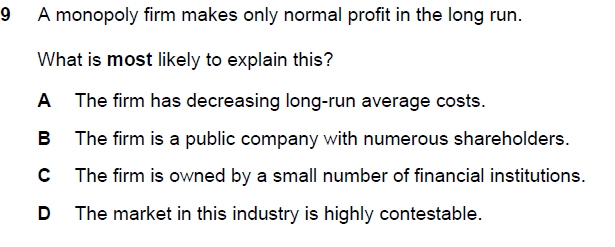The answer is (D). A contestable market is a market where the barriers to entry and exit are low. Since that is the case, it could be expected that there will be many firms that would join the industry over time. These firms would compete away the initial supernormal profits earned by the monopolist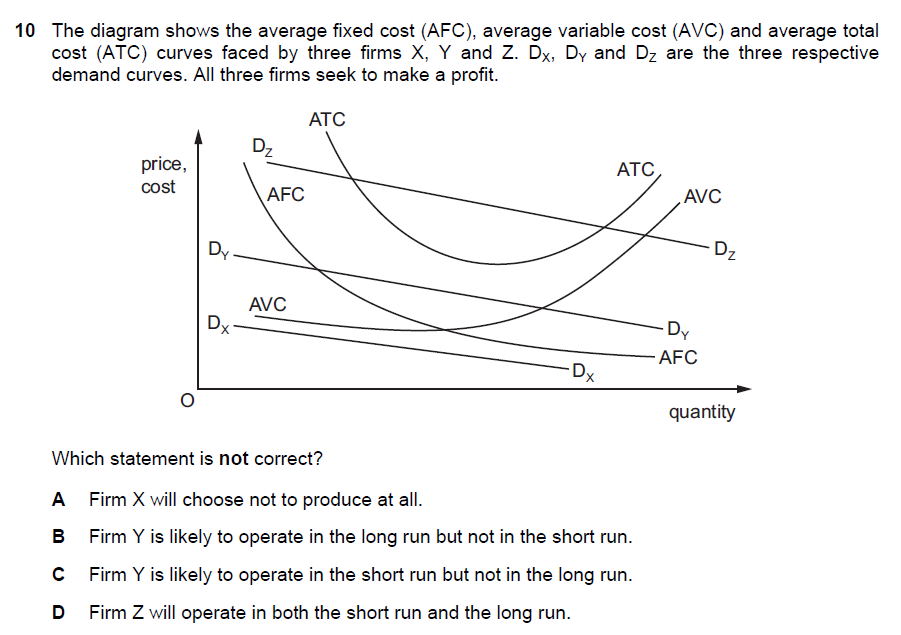The answer is (B). Firm Y is making a loss in the short-run. It will continue to operate since D = AR is above the AVC. However, if this were to persist, then it wouldn't make any business sense to continue the business into the long-run